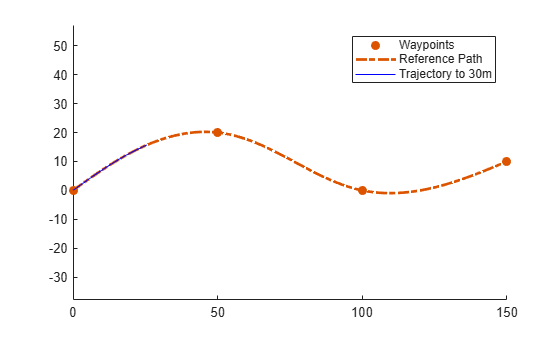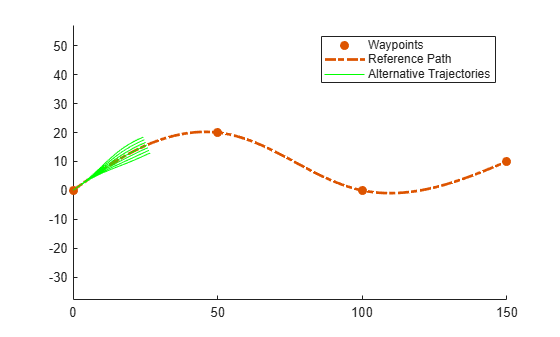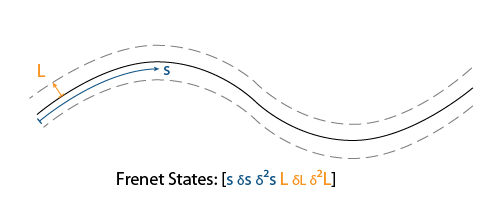# connect

Connect initial and terminal Frenet states

## Syntax

``frenetTrajectory = connect(connectorFrenet,initialState,terminalState,timeSpan)``
``[___,globalTrajectory] = connect(___)``

## Description

````frenetTrajectory = connect(connectorFrenet,initialState,terminalState,timeSpan)` connects the specified initial Frenet states to the specified terminal states over a span of time in seconds. This object function supports 1-to-n, n-to-1, or n-to-n pairwise trajectory connections.```

example

````[___,globalTrajectory] = connect(___)` returns the trajectories in global coordinates in addition to all arguments in the previous syntax.```

## Examples

collapse all

Generate alternative trajectories for a reference path using Frenet coordinates. Specify different initial and terminal states for your trajectories. Tune your states based on the generated trajectories.

Generate a reference path from a set of waypoints. Create a `trajectoryGeneratorFrenet` object from the reference path.

```waypoints = [0 0; ... 50 20; ... 100 0; ... 150 10]; refPath = referencePathFrenet(waypoints); connector = trajectoryGeneratorFrenet(refPath);```

Generate a five-second trajectory between the path origin and a point 30 m down the path as Frenet states.

```initState = [0 0 0 0 0 0]; % [S ds ddS L dL ddL] termState = [30 0 0 0 0 0]; % [S ds ddS L dL ddL] [~,trajGlobal] = connect(connector,initState,termState,5);```

Display the trajectory in global coordinates.

```show(refPath); hold on axis equal plot(trajGlobal.Trajectory(:,1),trajGlobal.Trajectory(:,2),'b') legend(["Waypoints","Reference Path","Trajectory to 30m"])```Create a matrix of terminal states with lateral deviations between –3 m and 3 m. Generate trajectories that cover the same arc length in 10 seconds, but deviate laterally from the reference path. Display the new alternative paths.

```termStateDeviated = termState + ([-3:3]' * [0 0 0 1 0 0]); [~,trajGlobal] = connect(connector,initState,termStateDeviated,10); clf show(refPath); hold on axis equal for i = 1:length(trajGlobal) plot(trajGlobal(i).Trajectory(:,1),trajGlobal(i).Trajectory(:,2),'g') end legend(["Waypoints","Reference Path","Alternative Trajectories"]) hold off```Specify a new terminal state to generate a new trajectory. This trajectory is not desirable because it requires reverse motion to achieve a lateral velocity of 10 m/s.

```newTermState = [5 10 0 5 0 0]; [~,newTrajGlobal] = connect(connector,initState,newTermState,3); clf show(refPath); hold on axis equal plot(newTrajGlobal.Trajectory(:,1),newTrajGlobal.Trajectory(:,2),'b'); legend(["Waypoint","Reference Path","New Trajectory"]) hold off```Relax the restriction on the longitudinal state by specifying an arc length of `NaN`. Generate and display the trajectory again. The new position shows a good alternative trajectory that deviates off the reference path.

```relaxedTermState = [NaN 10 0 5 0 0]; [~,trajGlobalRelaxed] = connect(connector,initState,relaxedTermState,3); clf show(refPath); hold on axis equal plot(trajGlobalRelaxed.Trajectory(:,1),trajGlobalRelaxed.Trajectory(:,2),'g'); hold off```## Input Arguments

collapse all

Frenet trajectory generator, specified as a `trajectoryGeneratorFrenet` object.

Initial Frenet states, specified as an n-by-6 numeric matrix. Each row of the matrix is a set of Frenet coordinates for the initial state of a trajectory in the form `[S dS ddS L dL ddL]`. The value of n must be equal to the number of rows in the `terminalState` argument or `1`.

Final Frenet states, specified as an n-by-6 numeric matrix. Each row of the matrix is a set of Frenet coordinates for the initial state of a trajectory in the form `[S dS ddS L dL ddL]`. The value of n must be equal to the number of rows in the `initialState` argument or `1`.

Time horizon for all trajectories, specified as a positive scalar in seconds. The generated trajectories are sampled evenly across this time span based on the TimeResolution property of the `trajectoryGeneratorFrenet` object specified in the `connectorFrenet` argument.

## Output Arguments

collapse all

Frenet trajectories between all initial and final states, returned as a structure array with these fields:

• `Trajectory`n-by-6 numeric matrix. Each row of the matrix is a set of Frenet coordinates for the initial state of a trajectory in the form `[S dS ddS L dL ddL]`.

• `Time` — Vector of positive scalars from `0` to `timeSpan` in seconds.This function supports 1-to-n, n-to-1, or n-to-n pairwise trajectory connections based on the number of rows of `initialState` and `terminalState`.

Global trajectories between all initial and final states, returned as structure or structure array with fields:

• `Trajectory`n-by-6 numeric matrix. Each row of the matrix is a set of global sates of the form ```[x y theta kappa dkappa s]```.

• `Time` — Vector of positive scalars from `0` to `timeSpan` in seconds.This function supports 1-to-n, n-to-1, or n-to-n pairwise trajectory connections based on the number of rows of `initialState` and `terminalState`.

## Extended Capabilities

### C/C++ Code GenerationGenerate C and C++ code using MATLAB® Coder™.

Introduced in R2020b